StatLect

# Moments of a random variable

This lecture introduces the notion of moment of a random variable.## Moment

The-th moment of a random variable is the expected value of its-th power.

Definition Letbe a random variable. Let. If the expected valueexists and is finite, thenis said to possess a finite-th moment andis called the-th moment of. Ifis not well-defined, then we say thatdoes not possess the-th moment.

The following example shows how to compute a moment of a discrete random variable.

Example Letbe a discrete random variable having support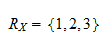and probability mass function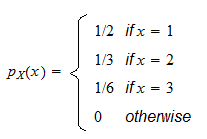The third moment ofcan be computed as follows: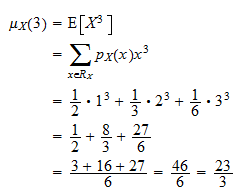## Central moment

The-th central moment of a random variableis the expected value of the-th power of the deviation offrom its expected value.

Definition Letbe a random variable. Let. If the expected value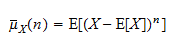exists and is finite, thenis said to possess a finite-th central moment andis called the-th central moment of.

The next example shows how to compute the central moment of a discrete random variable.

Example Letbe a discrete random variable having support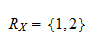and probability mass function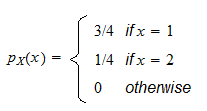The expected value ofis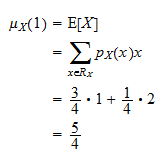The third central moment ofcan be computed as follows:## More details

The following subsections contain more details about moments.

### Multivariate generalization

A generalization of the concept of moment to random vectors is introduced in the lecture entitled Cross-moments.

### Computation

The moments of a random variable can be easily computed by using either its moment generating function, if it exists, or its characteristic function (see the lectures entitled Moment generating function and Characteristic function).

The book

Most of the learning materials found on this website are now available in a traditional textbook format.

Glossary entries
Share